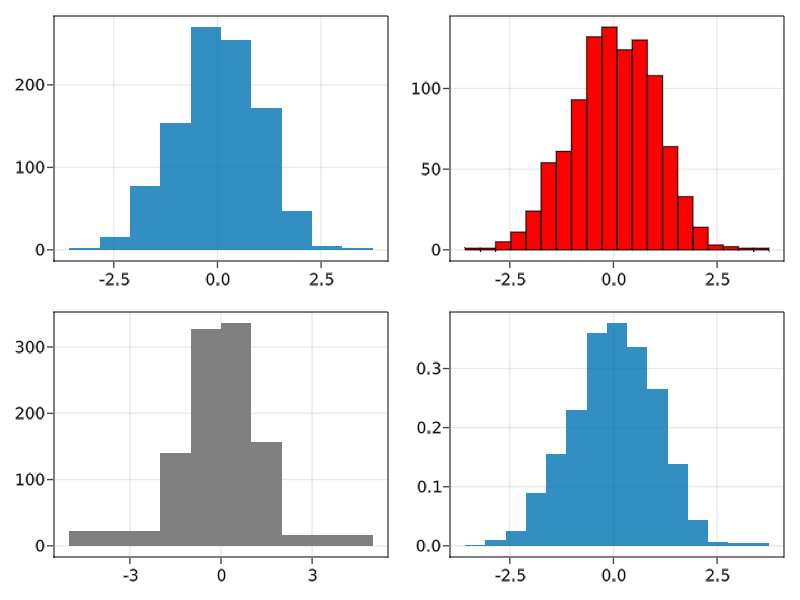# hist

Makie.histFunction
hist(values; bins = 15, normalization = :none)


Plot a histogram of values. bins can be an Int to create that number of equal-width bins over the range of values. Alternatively, it can be a sorted iterable of bin edges. The histogram can be normalized by setting normalization. Possible values are:

• :pdf: Normalize by sum of weights and bin sizes. Resulting histogram has norm 1 and represents a PDF.
• :density: Normalize by bin sizes only. Resulting histogram represents count density of input and does not have norm 1. Will not modify the histogram if it already represents a density (h.isdensity == 1).
• :probability: Normalize by sum of weights only. Resulting histogram represents the fraction of probability mass for each bin and does not have norm 1.
• :none: Do not normalize.

Color can either be:

• a vector of bins colors
• a single color
• :values, to color the bars with the values from the histogram

Attributes

Available attributes and their defaults for Combined{Makie.hist, T} where T are:

  bar_labels             "nothing"
bins                   15
color                  RGBA{Float32}(0.0f0,0.0f0,0.0f0,0.6f0)
cycle                  [:color => :patchcolor]
flip_labels_at         Inf
label_color            :black
label_font             "Dejavu Sans"
label_formatter        Makie.bar_label_formatter
label_offset           5
label_size             20
normalization          :none
over_background_color  MakieCore.Automatic()
over_bar_color         MakieCore.Automatic()
source

### Examples

using GLMakie

data = randn(1000)

f = Figure()
hist(f[1, 1], data, bins = 10)
hist(f[1, 2], data, bins = 20, color = :red, strokewidth = 1, strokecolor = :black)
hist(f[2, 1], data, bins = [-5, -2, -1, 0, 1, 2, 5], color = :gray)
hist(f[2, 2], data, normalization = :pdf)
f#### Histogram with labels

You can use all the same arguments as barplot:

using CairoMakie
CairoMakie.activate!()
hist(data, normalization = :pdf, bar_labels = :values,
label_formatter=x-> round(x, digits=2), label_size = 15,
strokewidth = 0.5, strokecolor = (:black, 0.5), color = :values)